##Circumcircle in trilinears

Start with a segment AB and invert it to A'B' w.r. to the circle c. Then |AB|/|A'B'| = d/d', where d and d' are the distances of the center O of c from the segments.
In fact, triangles OBA and OB'A' are similar etc....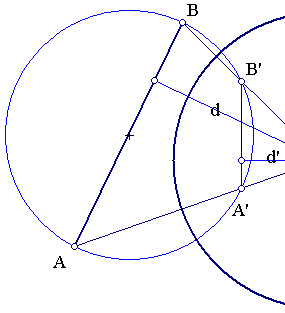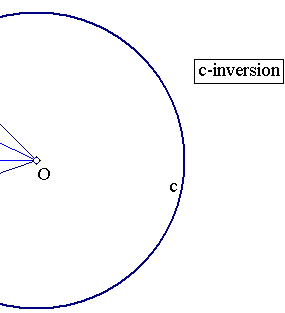Consider now a triangle ABC, its circumcircle f and the inversion on a circle c' with arbitrary radius and center O on F. f transforms to a line e and points A, B, C respectively to points A', B', C' on that line. Start from the vector equation A'B'+B'C'+C'A' = 0. Divide with the distance d of O from e to obtain A'B'/d + B'C'/d + C'D'd = 0. But using the previous exercise |A'B'|/d=|AB|/z, |B'C'|/d = |BC|/x, |C'A'|/d = |CA|/y. Hence substituting in the previous equation we get:
a/x + b/y + c/z = 0.
Where a=|BC|, b=|CA|, c=|AB|. This is the equation of the circumcircle f of ABC in trilinear coordinates w.r. to that triangle. Since the barycentric coordinates (x',y',z') are equal to (x*a,y*b,z*c), we get the equation of the circumcircle in barycentric coordinates:
a2/x' + b2/y' + c2/z' = 0. <==> a2*y'*z' + b2*z'*x' + c2*x'*y' = 0.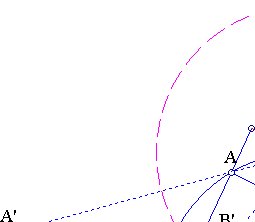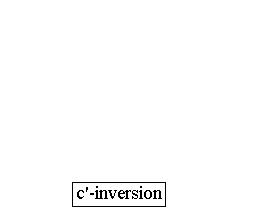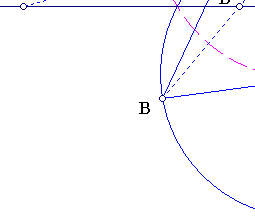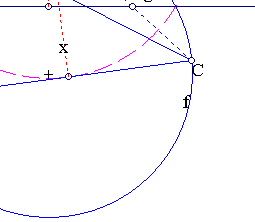AreaOfPedal.html
BarycentricsFormulas.html
BarycentricCoordinates.html
BarycentricCoordinates2.html
BarycentricCoordinates3.html
Isogonal_Conjugation.html
IsotomicConicOfLine.html
LineInTrilinears.html
Symmedian.html
TrilinearPolar.html
Trilinear_Polar.html
Tripole.html

### References

Casey, J. A Treatise on the Analytical Geometry of the Point, Line, Circle, and Conic Sections, Containing an Account of Its Most Recent Extensions, with Numerous Examples, 2nd ed. rev. enl. . Dublin, Hodges, Figgis, & Co., 1893, p. 128
Yiu, P. GeometryNotes020402 (pdf). http://www.math.fau.edu/yiu/GeometryNotes020402.pdf p. 63